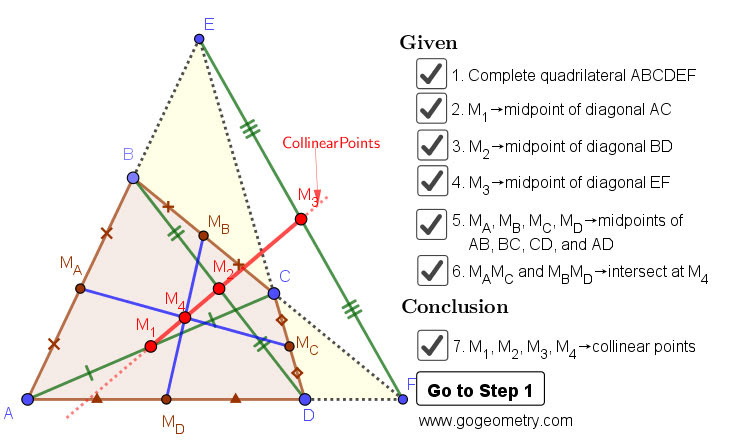## Sunday, March 15, 2020

### Dynamic Geometry 1462: Newton-Line, Newton-Gauss Line, Complete Quadrilateral, Midpoints of Sides and Diagonals

Interactive step-by-step animation using GeoGebra. Post your solution in the comment box below.
Level: Mathematics Education, High School, Honors Geometry, College.

Details: Click on the figure below.#### 1 comment:

1.From the results of Problem 1460, M1,M2 and M3 are collinear. M1,M2 and M4 are collinear for any quadrilateral.
Proof:MaMbMcMd is parallelogram, hence MaMc and MbMd bisect each other, also M4 is midpoint of MaMc and MbMd. Similarly MaM2McM1 is also parallelogram. Diagonal M1M2 must pass through midpoint of MaMc which is M4. Hence M1,M2 and M4 are collinear, since M1,M2 and M3 are also collinear. We have M1,M2,M3 and M4 collinear.